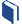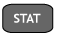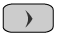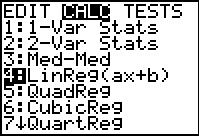# Exploring Other Function Models

###Resources for this lesson:

> Glossary> Calculator Resources> Teacher Resources: Instructional Notes## Test and Confirm

Function Families
Cubic Function
Quartic Function
Power Function
Logarithmic Function
Logistic Function
Trigonometric Function

For each of the function families above, the graphing calculator will calculate a regression equation.  To access these regression equations, presson your graphing calculator.  You will see this screen:The numbers or letters in the list correspond to the following regression equations:

4:  Linear Regression